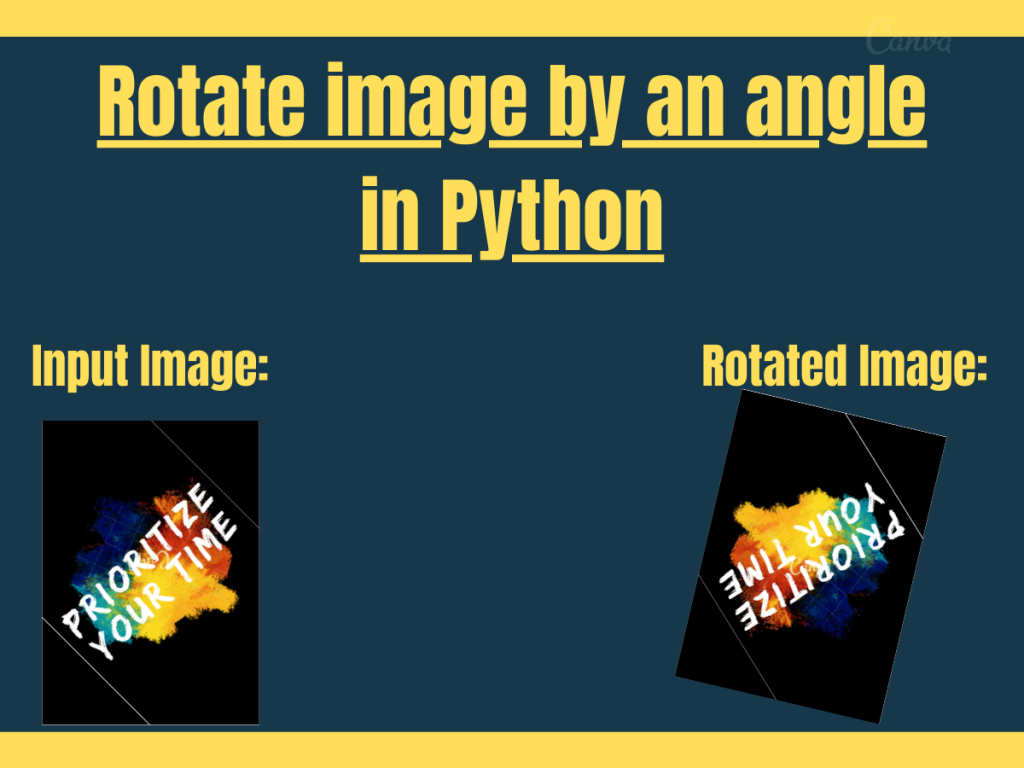# 2 ways to rotate an image by an angle in PythonHey, folks! In this article, we will be unveiling ways to rotate an image by an angle in Python.

By rotating an image we mean that the image is rotated by its centre to a specified degree.

## Technique 1: Python Image Library(PIL)

`PIL -- Python Image Library` is a module that contains in-built functions to manipulate and work with image as an input to the functions.

PIL provides in-built `image.rotate(angle) function` to rotate an image by an angle in Python.

Syntax:

```image.rotate(angle)
```

In order to load an image or pass an image to the rotate() function, we need to use the below snippet of code:

```Image.open(r"URL of the image")
```

We need to use the below snippet of code to display the image:

```image.show()
```

Example 1:

```from PIL import Image

img = Image.open(r"C:\Users\HP\OneDrive\Desktop\Penskull Education.png")

rotate_img= img.rotate(125)

rotate_img.show()

```

In the above snippet of code, we have rotated the input image by an angle of 125 degree.

Input Image:

Output:

Example 2:

```from PIL import Image

img = Image.open(r"C:\Users\HP\OneDrive\Desktop\Penskull Education -- 01.png")

rotate_img= img.rotate(45)

rotate_img.show()

```

In this example, the image is being rotated by an angle of 45 degree.

Output:

## Technique 2: OpenCV to rotate an image by an angle in Python

`Python OpenCV` is a module that deals with real-time applications related to computer vision. It contains a good number of built-in functions to deal with images as input from the user.

OpenCV works well with another image processing library named ‘`imutils`‘ to manipulate and work with images.

The `imutils.rotate() function` is used to rotate an image by an angle in Python

Syntax:

```imutils.rotate(image, angle=angle)
```

Syntax: To read an image as input using OpenCV

```cv2.imread(r"image path/URL")
```

Syntax: To display the image using OpenCV

```cv2.imshow("output--msg",image)
```

Example:

```import cv2
import imutils

rot = imutils.rotate(image, angle=45)
cv2.imshow("Rotated", rot)
cv2.waitKey(0)
```

Output: